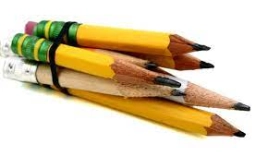# 2 pens

Two pens and 3 pencils cost Rs. 26, 3 pens and 5 pencils cost Rs. 40. What is the cost of one pen and one pencil?

a =  10
b =  2

### Step-by-step explanation:

2a+3b=26
3a+5b=40

2·a+3·b=26
3·a+5·b=40

2a+3b = 26
3a+5b = 40

Pivot: Row 1 ↔ Row 2
3a+5b = 40
2a+3b = 26

Row 2 - 2/3 · Row 1 → Row 2
3a+5b = 40
-0.33b = -0.67

b = -0.66666667/-0.33333333 = 2
a = 40-5b/3 = 40-5 · 2/3 = 10

a = 10
b = 2

Our linear equations calculator calculates it.Did you find an error or inaccuracy? Feel free to write us. Thank you!

Tips for related online calculators
Do you have a system of equations and looking for calculator system of linear equations?